## Sunday, July 23, 2017

### The CDF of the Gamma distribution in GAMS

For a model (1) I needed to calculate the cumulative distribution function $$F(x)$$ of the Gamma distribution. There is no uniform consensus:  the Gamma distribution has different parametrizations. I use the $$k, \theta$$ parametrization from (2). This would yield (3):

 $F(x) = \gamma( \frac{x}{\theta},k)$

where $$\gamma(x,a)$$ is the incomplete Gamma function defined by:

 $\gamma(x,a) = \frac{1}{\Gamma(a)} \int_0^x t^{a-1}e^{-t} dt$

The function $$\Gamma(x)$$ is the (complete) Gamma function:

 $\Gamma(x) = \int_0^{\infty} t^{x-1} e^{-t} dt$

Unfortunately there a few alternative definitions of the incomplete Gamma function. In (2) the following definition is used:

 $\gamma(s,x) = \int_0^x t^{s-1} e^{-t} dt$

So watch out: different definitions are being used.

As  a result we can implement the CDF of the Gamma distribution with parameter $$k$$ and $$\theta$$ as follows (2):

GammaReg(x/theta,k)

There is also a more obscure way to calculate this using an extrinsic function. The syntax is unfortunately needlessly complicated and the documentation is really, really bad (4).

 scalars  k / 0.363 /  theta / 27.863 /  x / 1.5 /  p;p = gammareg(x/theta,k);display p;$FuncLibIn stodclib stodclibfunction cdfGamma / stodclib.cdfGamma /;p = cdfGamma(x,k,theta);display p; #### References 1. Modeling Flood Damages, http://yetanothermathprogrammingconsultant.blogspot.com/2017/07/modeling-flood-damages.html 2. Gamma Distribution, https://en.wikipedia.org/wiki/Gamma_distribution 3. New Special Functions in GAMS, http://www.amsterdamoptimization.com/pdf/specfun.pdf 4. Stochastic Library, https://www.gams.com/latest/docs/userguides/userguide/_u_g__extrinsic_functions.html#UG_ExtrinsicFunctions_StochasticLibrary ### no loops please In (1) a piece of matlab code is presented: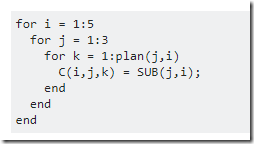There may be better way to formulate this in Matlab. In many languages, including Matlab, R and GAMS loops are considered bad if there are alternatives. To be honest, I don’t see immediately how this particular fragment can be vectorized in Matlab. The suggested GAMS version is: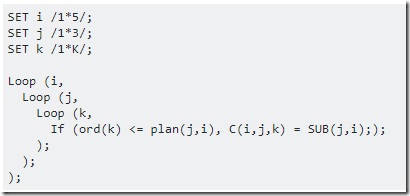I strongly disagree. I believe this can be formulated without loops as:  c(i,j,k)$(ord(k)<=plan(j,i)) = sub(j,i); 

Also notice that MATLAB will store this in a fully allocated or dense matrix (unless explicitly using a sparse matrix) while GAMS always stores things in a sparse format.

#### References

1. Generate Data using for loop in GAMS. https://stackoverflow.com/questions/45167648/generate-data-using-for-loop-in-gams

## Tuesday, July 18, 2017

### Ubuntu Bash on Windows

Windows 10 has a beta feature: Bash.exe with an Ubuntu Linux subsystem (see (1) and (2)), also known as WSL (Windows Subsystem for Linux). This will allow you to run Linux command line tools from within Windows. This is not the same as running Linux in a Virtual Machine (VM) using virtualization software like VirtualBox or VMware. It is also different from Cygwin or MINGW which is a windows port of GNU tools. In the case of  WSL, real, unmodified Ubuntu Linux binaries are executed.There is a single Linux instance, so when invoking bash.exe several times, you talk to the same instance:

The main issue I noticed is that some networking commands (like ping) requires bash.exe to be started as administrator.

#### References

1. Jack Hammons, Bash on Ubuntu on Windows, https://msdn.microsoft.com/commandline/wsl
3. VirtualBox, https://www.virtualbox.org/
4. VMware, https://www.vmware.com/
5. Cygwin, https://www.cygwin.com/
6. MINGW, Minimalist GNU for Windows, http://www.mingw.org/

## Monday, July 17, 2017

### Modeling flood damages

While working on a investment planning model to combat damages resulting from flooding, I received the results from a rainfall model that calculates damages as a result of excessive rain and flooding. The picture the engineers produced looks like:

This picture has the scenario on the x-axis (sorted by damage) and the damages on the y-axis. This picture is very much like a load-duration curve in power generation.

For a more “statistical” picture we can use standard histogram (after binning the data):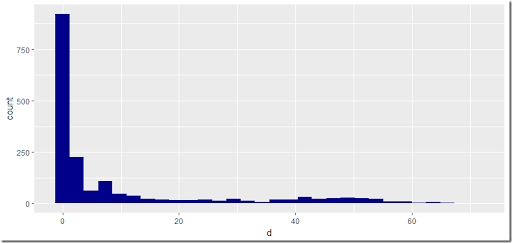##### Gamma distribution

We can use standard techniques to fit a distribution. When considering a Gamma distribution (1), a simple approach is the method of moments. The mean and the variance of the Gamma distribution with parameters $$k$$ and $$\theta$$ are given by:

 \begin{align} &E[X] = k \cdot \theta\\ & Var[X] = k \cdot \theta^2 \end{align}

Using sample mean $$\mu$$ and standard deviation $$\sigma$$, we can solve:

 \begin{align} & k \cdot \theta = \mu\\ & \sqrt{k} \cdot \theta = \sigma \end{align}

This can be easily solved numerically and it actually seems to work:

##### Weibull distribution

An alternative distribution that is sometimes suggested is the Weibull distribution (2).  The method of moments estimator for the Weibull distribution with parameters $$\lambda$$ and $$k$$ can be found by solving the system:

 \begin{align} & \lambda \Gamma(1+1/k) = \mu\\ &\lambda \sqrt{\Gamma(1+2/k)+\left(\Gamma(1+1/k)\right)^2} = \sigma \end{align}
I was unable to get a solution from this: solvers failed miserably on this.

An alternative approach would be to use an MLE (Maximum Likelihood Estimation) technique. This yields a system of equations:

 \begin{align} & \lambda^k = \frac{1}{n}\sum_{i=1}^n x_i^k\\ &k^{-1} = \frac{\sum_{i=1}^n x_i^k \ln x_i}{\sum_{i=1}^n x_i^k} – \frac{1}{n}\sum_{i=1}^n \ln x_i \end{align}
Note that we can solve the second equation first to solve for $$k$$ and then calculate $$\lambda$$ using the first equation. A solver like CONOPT will do this automatically by recognizing the triangular structure of this system. Note that our data set contains many $$x_i=0$$. These are replaced by $$x_i=0.001$$ so we can take the logarithm.

This gives is a very similar picture:

##### References
1. Gamma distribution, https://en.wikipedia.org/wiki/Gamma_distribution
2. Weibull distribution, https://en.wikipedia.org/wiki/Weibull_distribution

## Tuesday, July 11, 2017

### Rectangles: no-overlap constraints

The question of how we can formulate constraints that enforce rectangles not to overlap comes up regularly (1). There are basically four cases to consider when considering two rectangles $$i$$ and $$j$$: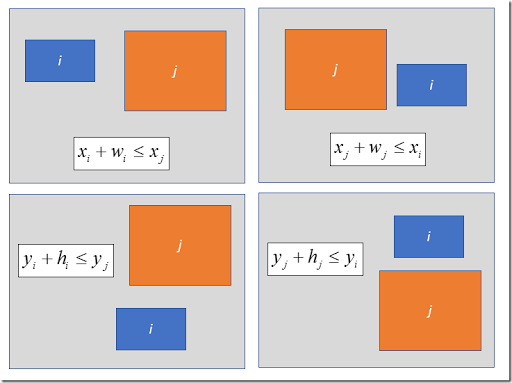In the picture above, we indicate by $$(x_i,y_i)$$ the left-lower corner of a rectangle (these are typically decision variables). The height and the width are $$h_i$$ and $$w_i$$ (these are typically constants). From the above, we can formulate the “no-overlap”  constraints as:

 \bbox[lightcyan,10px,border:3px solid darkblue] {\begin{align}&x_i+w_i \le x_j & \text{or} \\&x_j+w_j \le x_i & \text{or} \\&y_i+h_i \le y_j & \text{or} \\&y_j+h_j \le y_i \end{align} }

The “or” condition can be modeled with the help of binary variables $$\delta$$ and big-M constraints:

 \bbox[lightcyan,10px,border:3px solid darkblue] {\begin{align}&x_i+w_i \le x_j + M_1 \delta_{i,j,1}\\&x_j+w_j \le x_i + M_2 \delta_{i,j,2}\\&y_i+h_i \le y_j + M_3 \delta_{i,j,3}\\&y_j+h_j \le y_i+ M_4 \delta_{i,j,4}\\&\sum_k \delta_{i,j,k} \le 3\\& \delta_{i,j,k} \in \{0,1\} \end{align} }

The binary variables make sure at least one of the constraints is active (not relaxed).

If we have multiple rectangles we need to compare all combinations, i.e. we generate these constraints for  $$\forall i,j$$. Except: we can not compare a rectangle with itself. A first approach would be to generate the above constraints for all $$i\ne j$$. This is correct, but we can apply an optimization. Note that we actually compare things twice: if rectangles $$i$$ and $$j$$ are non-overlapping then we don’t need to check the pair of rectangles $$j$$ and $$i$$. That means we only need constraints and variables $$\delta_{i,j,k}$$ for $$i<j$$.

It is important to make constants $$M_k$$ as small as possible. In general we have a good idea about good values for these $$M_{k}$$’s. E.g. consider the case where we need to pack boxes in a container (2):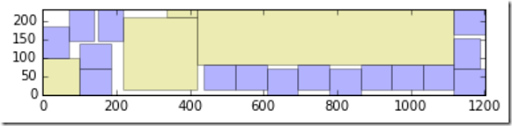If the container has length and width $$H$$ and $$W$$ then we can easily see: $$M_1=M_2=W$$ and $$M_3=M_4=H$$.

##### References
1. How to write this constraint in LPSolve?, https://stackoverflow.com/questions/44858353/how-to-write-this-constraint-in-lp-solver-logical-and-and-doesnot-exist
2. Filling up a container with boxes, http://yetanothermathprogrammingconsultant.blogspot.com/2016/06/filling-up-container-with-boxes.html

## Monday, July 10, 2017

### GLPK was used in the proof of the 300 year old Kepler conjecture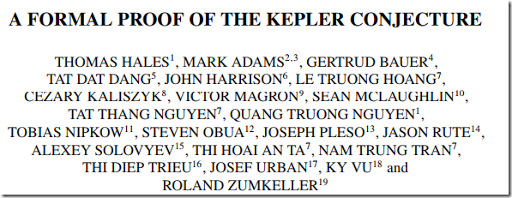A lot of authors.

Apparently 43,078 LPs had to be solved.

##### References
1. Thomas Hales e.a., A Formal Proof of the Kepler Conjecture, Forum of mathematics, Pi (2017), vol 5, e2, https://www.cambridge.org/core/services/aop-cambridge-core/content/view/78FBD5E1A3D1BCCB8E0D5B0C463C9FBC/S2050508617000014a.pdf/formal_proof_of_the_kepler_conjecture.pdf
2. Original message appeared in the Help-GLPK mailing list,  https://lists.gnu.org/archive/html/help-glpk/2017-07/msg00001.html